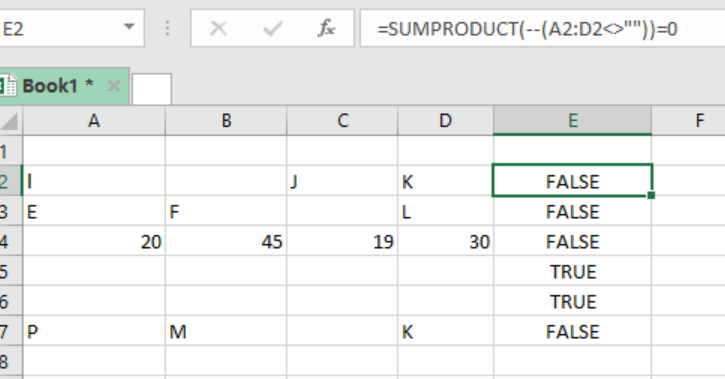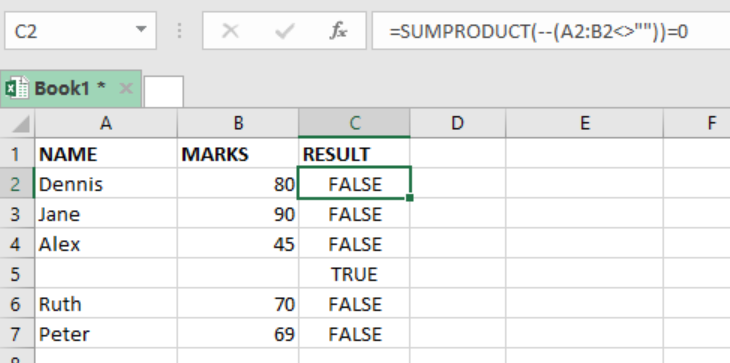Go Back

# All cells in range are blank

We can use the SUMPRODUCT function in Excel to check or test if all cells in a range are blank. If all cells in the specified range are empty, the function will return TRUE. But if not all cells are empty, then it will return a FALSE. This article provides a step-by-step guide on how to test if all cells are empty using the SUMPRODUCT function based on some logical tests.Figure 1: Final result

## Syntax of the formula

`=SUMPRODUCT(- - (range<>””))=0`

## Explanation of the use case

The SUMPRODUCT function returns a TRUE if cells in range are blank or empty, and FALSE if not all cells are empty. In the example above, the formula in cell E2 is as below:

`=SUMPRODUCT(--(A2:D2<>””))=0`

The inner part of the SUMPRODUCT formula, i.e. ‘- -(A2:D2<>””) tests each cell in the given range.

Inside the parentheses, we have A2:D2<>””, which looks like: {TRUE, FALSE, TRUE}

Outside the parentheses we have the double hyphen, which is responsible for converting the TRUE FALSE into 1s and 0s, i.e. {1,0,1}

Remember that 1s in this case represents cells that are not empty. The SUMPRODUCT function will then multiply the values and return the expected value, either TRUE or FALSE.

Usually, the result of the multiplication will be greater than zero. But we know that not every cell in the range will be empty. So we shall have to add =0 at the end of the formula in order to force it return TRUE if the cells are empty, and FALSE if not empty.

## ExampleFigure 2: Finding empty cells in a class list

The table above shows a list of students’ names and their marks. We want to find which cells are empty in the range.

-In column C, we specify the result. In cell C2, we specify our formula,

`=SUMPRODUCT(- - (A2:B2<>””))=0`

-We then press Enter to get the result in cell C2.

-We then copy down the formula to get the results for the other cells.

## Instant Connection to an Expert through our Excelchat Service

Most of the time, the problem you will need to solve will be more complex than a simple application of a formula or function. If you want to save hours of research and frustration, try our live Excelchat service! Our Excel Experts are available 24/7 to answer any Excel question you may have. We guarantee a connection within 30 seconds and a customized solution within 20 minutes.

### Did this post not answer your question? Get a solution from connecting with the expert.

Another blog reader asked this question today on Excelchat:
Here are some problems that our users have asked and received explanations onAppend multiple ranges in to one range & skip blank cells.
Solved by E. W. in 16 minsI have had 3 experts help me and havnt gotten a working solution yet for my issue. I am trying to move all the values that are not blank in a range and list them in another range with no blank cell.
Solved by F. H. in 30 minsAppend range while skipping blank cells
Solved by E. W. in 11 mins